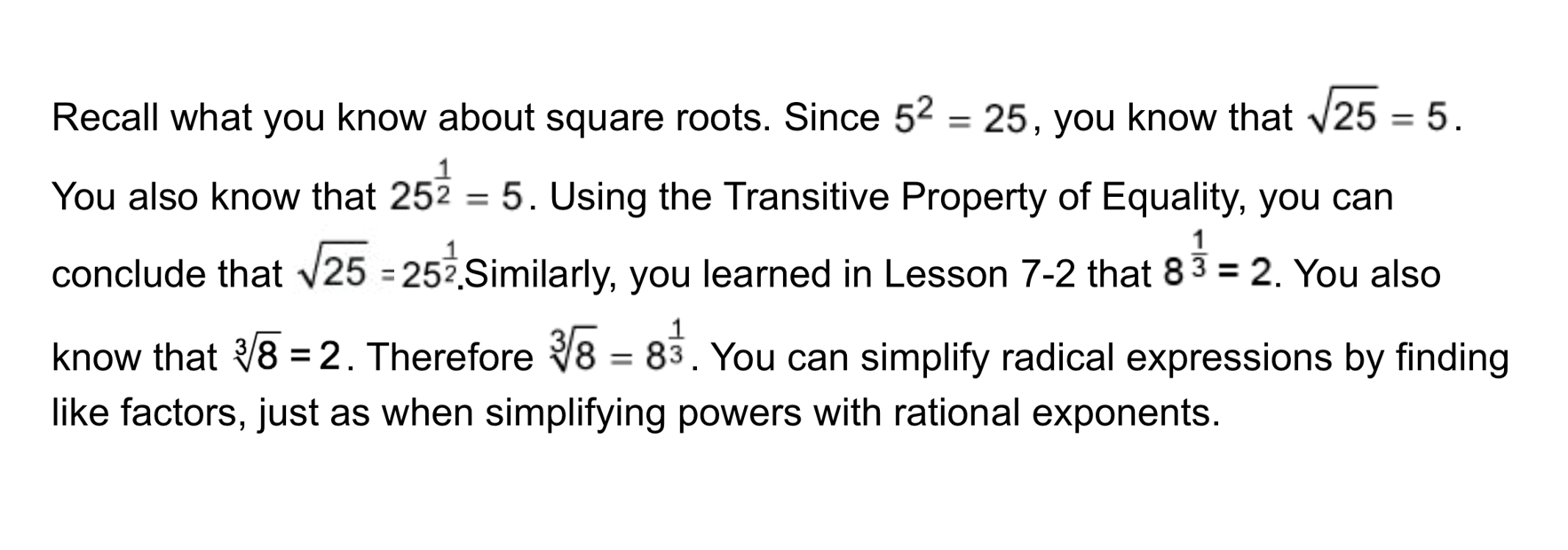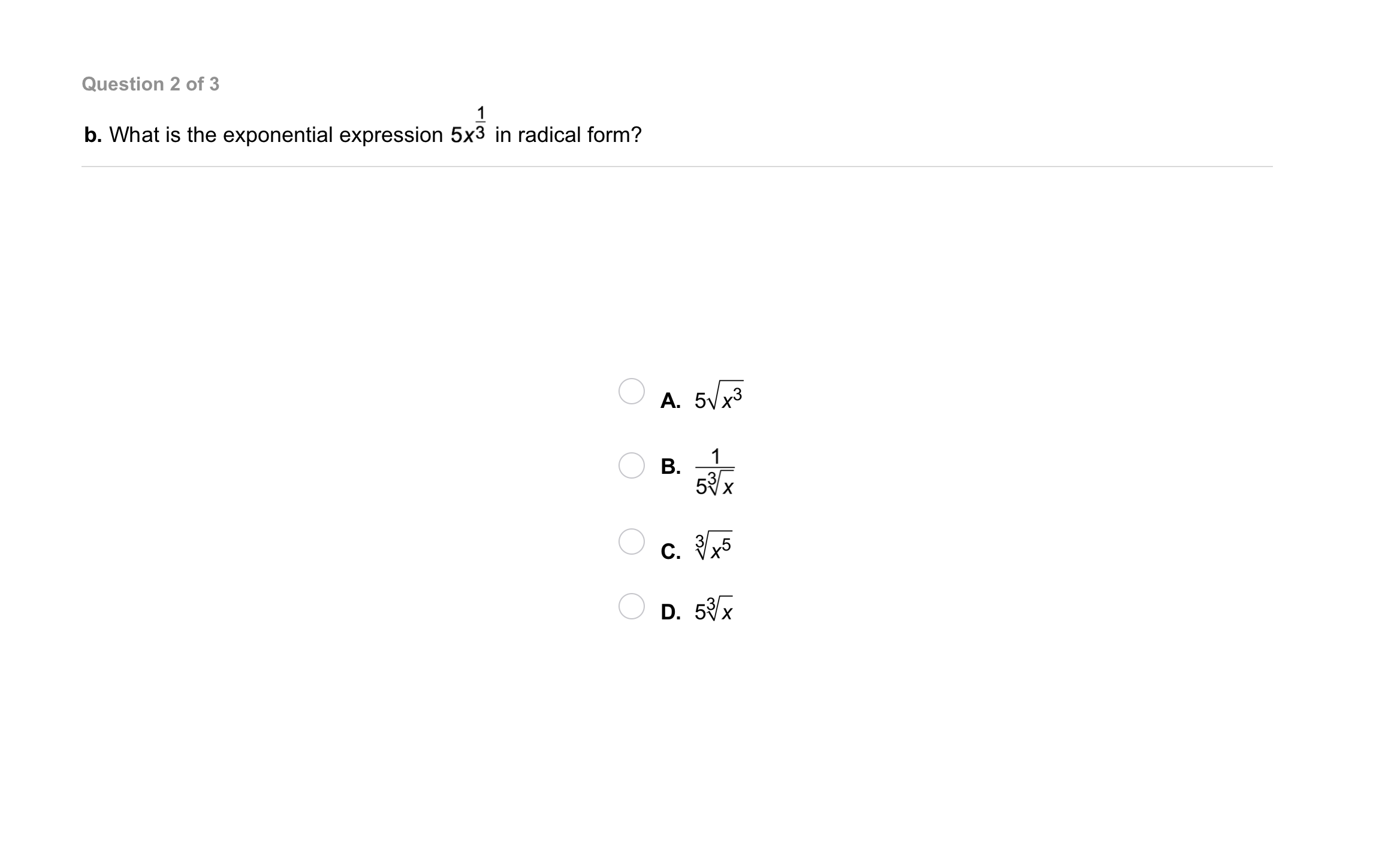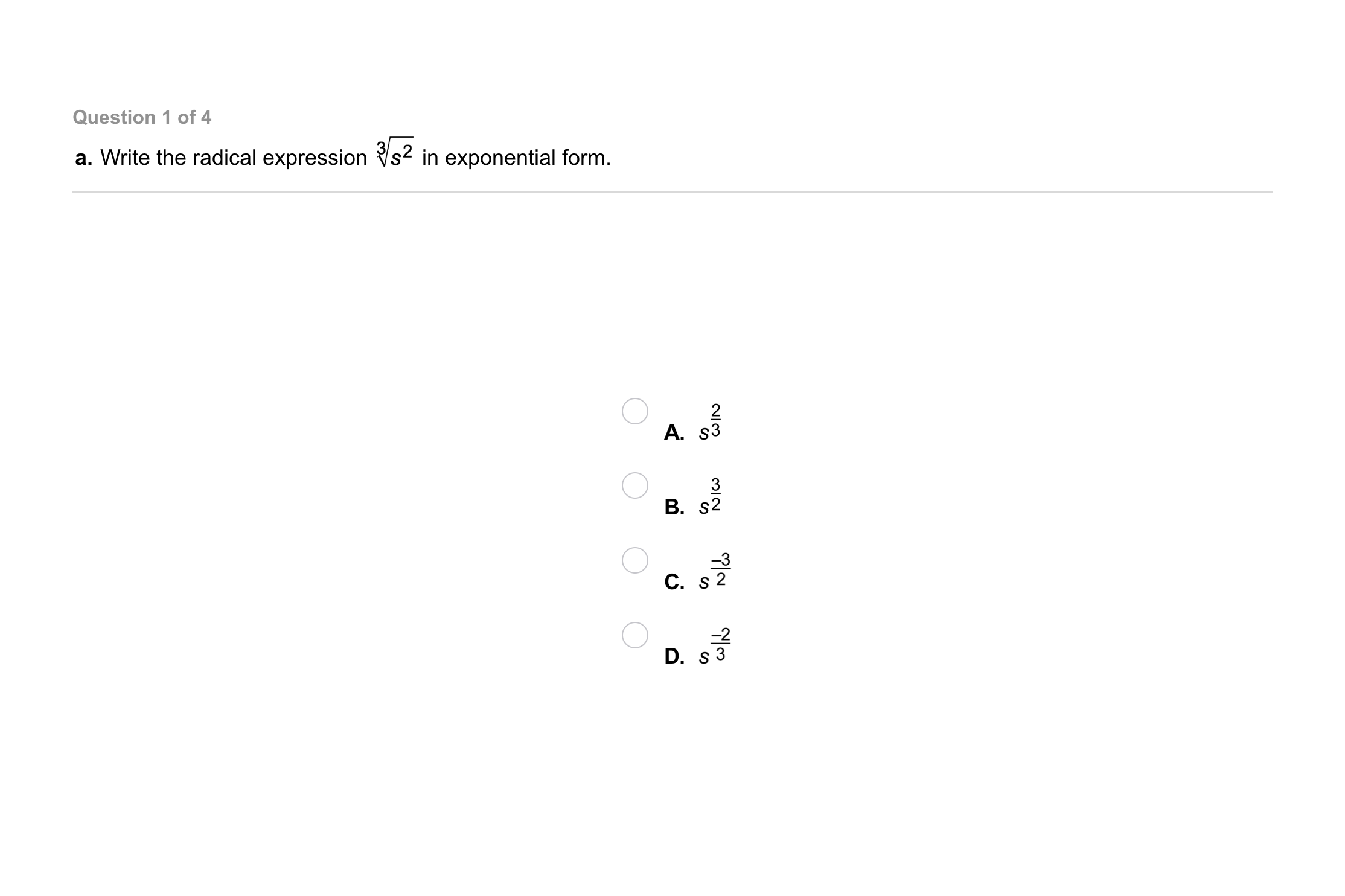Algebra 1 7-5 Guided Practice: Rational Exponents and Radicals
starstarstarstarstarstarstarstarstarstar
by Matthew Richardson
| 13 Questions1
1
10
Solve It! The figure is made up of squares. The side of a larger square is bisected by the vertices of the square that is one size smaller. What are the values of a, b, and c? Hint: The hypotenuse of an isosceles right triangle equals the smaller side times the square root of 2.2
2
10
Problem 1 Got It?
A
B
C
D3
3
10
Problem 1 Got It?
A
B
C
D4
4
10
Problem 1 Got It?
A
B
C
D5
5
10
Problem 1 Got It?
A
B
C
D6
6
10
Problem 2 Got It?
A
B
C
D7
7
10
Problem 2 Got It?
A
B
C
D8
8
10
Problem 2 Got It?
A
B
C
D9
9
10
Problem 3 Got It?
A
B
C
D10
10
10
Problem 3 Got It?
A
B
C
D11
11
10
Problem 3 Got It?
A
B
C
D12
12
10
Problem 3 Got It?
A
B
C
D13
13
10
Problem 4 Got It?
A
B
C
D